# Test: Equation Of Plane

## 10 Questions MCQ Test Mathematics (Maths) Class 12 | Test: Equation Of Plane

Description
This mock test of Test: Equation Of Plane for JEE helps you for every JEE entrance exam. This contains 10 Multiple Choice Questions for JEE Test: Equation Of Plane (mcq) to study with solutions a complete question bank. The solved questions answers in this Test: Equation Of Plane quiz give you a good mix of easy questions and tough questions. JEE students definitely take this Test: Equation Of Plane exercise for a better result in the exam. You can find other Test: Equation Of Plane extra questions, long questions & short questions for JEE on EduRev as well by searching above.
QUESTION: 1

### The equation of the plane passing through the point (3, – 3, 1) and perpendicular to the line joining the points (3, 4, – 1) and (2, – 1, 5) is:​

Solution:

The equation of the plane passing through the point (3, – 3, 1) is:
a(x – 3) + b(y + 3) + c(z – 1) = 0 and the direction ratios of the line joining the points
(3, 4, – 1) and (2, – 1, 5) is 2 – 3, – 1 – 4, 5 + 1, i.e., – 1, – 5, 6.
Since the plane is perpendicular to the line whose direction ratios are – 1, – 5, 6, therefore, direction ratios of the normal to the plane is – 1, – 5, 6.
So, required equation of plane is: – 1(x – 3) – 5(y + 3) + 6(z – 1) = 0
i.e., x +  5y – 6z + 18 = 0.

QUESTION: 2

### The equation of the plane, which is at a distance of 5 unit from the origin and has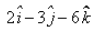as a normal vector, is:

Solution:

x = 3i - 2j - 6k
|x| = ((3)2 + (2)2 + (6)2)
|x| = (49)½
|x| = 7
x = x/|x|
= (3i - 2j - 6k)/7
The required equation of plane is r.x = d
⇒  r.(3i - 2j - 6k)/7 = 5
⇒  r.(3i - 2j - 6k) = 35

QUESTION: 3

### The Cartesian form of the equation of the plane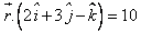is:

Solution:Let r = xi + yj + zk
(xi + yj + zk) . (2i + 3j - k) = 10
2x + 3y - k = 10

QUESTION: 4

The length of the perpendicular from the origin to the plane 3x + 2y – 6z = 21 is:​

Solution:

The given point is P(0,0,0) and the given line is 3x+2y-6z-21=0
Let d be the length of the perpendicular from P(0,0) to the line 3x+2y-6z-21=0
Then, d= |3*0 + 2*0 + (-6)*0 -21|/[(3)^2 + (2)2 + (-6)2]1/2
= |-21|/7
= 3

QUESTION: 5

The slope intercept form of the linear equation is

Solution:
QUESTION: 6

If l, m, n are the direction cosines of the normal to the plane and p be the perpendicular distance of the plane from the origin, then the equation of the plane is:

Solution:

Let P(x, y, z) be any point on the plane.
OP = r = xi + yj +zk
Let l,m,n be the direction of cosine of n, then
n - li + mj + nk
As we know that r.n = d
(xi + yj + zk)(li + mj + nk) = d
i.e. lx + my + nz = d
this is the cartesian equation of plane in normal form.

QUESTION: 7

If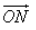is the normal from the origin to the plane, and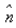is the unit vector along. P(x, y, z) be any point on the plane and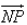is perpendicular to. Then

Solution:

NP is perpendicular to ON
NP.ON = |NP||ON| cosθ  (θ = 90deg)
|NP||ON|cos90deg
⇒ 0

QUESTION: 8

The length of the perpendicular from the origin to the plane 2x – 3y + 6z = 21 is:​

Solution:
QUESTION: 9

The angle between two lines whose direction ratios are 1,2,1 and 2,-3,4 is:​

Solution:

(1,2,1) and (2, -3, 4)
Cosθ = ​(a1b1+a2b2+a3b3]/[(a1)2+(a2)2 + (a3)2)]1/2 [(b1)2 + (b2)2 + (b3)2]1/2
Cosθ = [(1)(2) + (2)(-3) + (1)(4)]/ [(1)^2 + (2)^2 + (1)2]½ [(2)2 + (-3)2 + (4)2]½
Cosθ = [2 - 6 + 4]/[1 + 4 + 1]½ [4 + 9 + 16]½
Cosθ = 0
θ = 90deg

QUESTION: 10

The equation of the plane passing through the points (2, 1, 0), (3, – 2, – 2) and (3, 1, 7) is:​

Solution:

We know that, the equation of a plane passing through three non-collinear points (x1. y1, z1), (x2 , y2 , z2) and (x3, y3, z3)
{(x-x1) (y-y1) (z-z1)} {(x2-x1) (y2-y1) (z2-z1)} {(x3-x2) (y3-y2) (z3 - z2)}
= {(x-2) (y-1) (z-0)} {(3-2) (-2-1) (-2-0)} {(3-2) (1-1) (7-0)} = 0
= {(x-2) (y-1) (z)} {(1) (-3) (-2)} {(1) (0) (7)}
=> (x-2)(-21) - (y-1)(9) + z(3) = 0
7x + 3y - z = 17